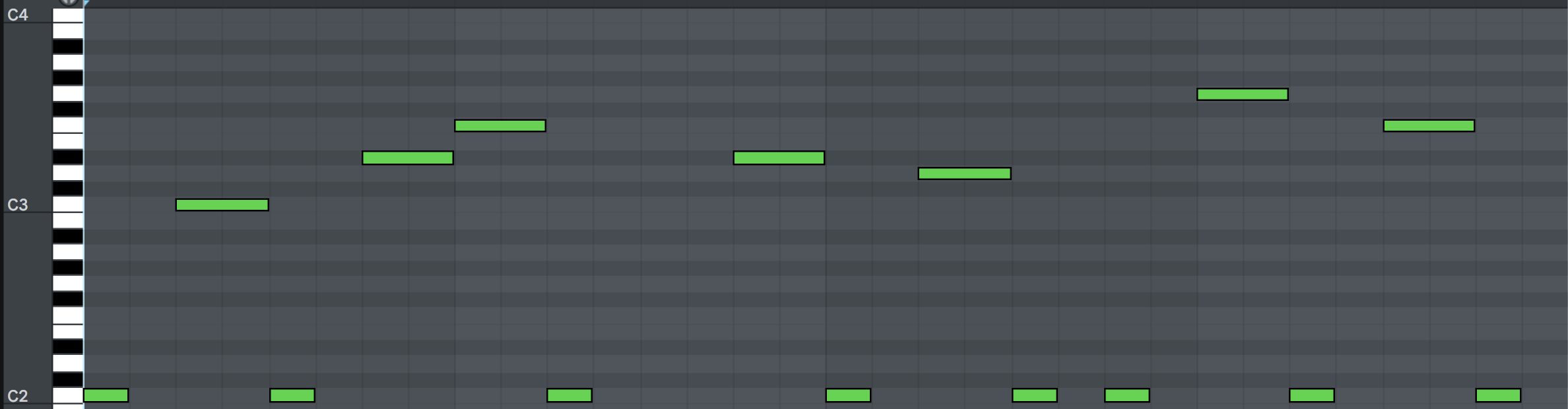### Riff

Scribbletune can be used to generate a commonly used pattern for riffs in trance music.

Before we go on, here’s a sample of what we are gonna end up creating using Scribbletune.

If you d like to watch a video on how to do this,

As you can imagine, this is a commonly used technique to create riffs. You randomly click some of the steps in a 16 beat pattern and move them around while sticking to a particular scale. Then, on a lower octave, add notes to the missing steps on a particular key to act as the counter point notes. This works great and quickly creates a nice little riff that looks a bit like this,Instead of doing it in a DAW, you can create a JavaScript function that does this and allow it to accept some things dynamically such as “key”, “scale” and “chord degrees” to use as the progression. If you’d like to skip the explanation and directly use the script, then please click here

#### Pattern

Since we start by clicking around randomly in a DAW, we can easily create a JavaScript function that does this for us. We can use the `x` character to act as our counterpoint root note and `R` as our offbeat note that acts as the melodic counterpart of our riff.

``````const getRandomPattern = function (count) {
let str = '[x-]R';
for (let i = 1; i < (count || 8); i++) {
str += Math.round(Math.random()) ? '[x-]R' : 'R[x-]';
}

return str;
}
``````

Here we are starting with `[x-]R` as I would like to have the root note of our riff to have a “note on” event every time the riff starts. From then on, we are randomly alternating between `[x-]R` and `R[-x]` instead of `[x-]` and `R` to avoid creating a pattern that is too sparse. The reason we are using `[x-]` instead of `x` is to subdivide that note so that the `x` plays for half it’s duration which could be interesting for the riff as I cannot do palm muting with JavaScript (yet). The function will produce something like,

``````[x-]RR[x-][x-]R[x-]R[x-]R[x-]R[x-]RR[x-]
``````

And it will create a slightly different pattern each time.

#### Random notes

Next, we’ll create a clip and set the notes to be used. We’ll also provide a set of notes for Scribbletune to choose from, for the `R` character. We can simply use the higher octave of our root note. So something like,

``````const clip = scribble.clip({
notes: 'D2',
randomNotes: 'D3',
pattern: getRandomPattern(),
subdiv: '16n',
});
``````

At this point we are pretty much ready to render a MIDI clip of our riff.

``````scribble.midi(clip, 'riff.mid');
``````

This will create a simple riff that could very well be used as a bassline at least. It will alternate between the D2 and the D3 note in our riff pattern, taking D2 for every `x` and D3 for every `R`. To make this a bit more interesting, we can set the pattern like this to get a riff that sounds a bit more “complete”,

``````pattern: getRandomPattern() + getRandomPattern(),
``````

#### Melody

What we have so far can pass for a riff, but very soon we would like to spice it up a bit. Let’s provide more notes to the `randomNotes` param. Instead of passing a single `D3` note, we could pass it a chord progression instead.

``````randomNotes: scribble.arp(
scribble.getChordsByProgression('D2 minor', 'ii iii')
),
``````

To take it up just one notch up, we could generate 2 clips, Clip A and Clip B. For Clip A, we will use the `ii` and `iii` chord degree and for Clip B, we’ll use the `iii` and `v` chord degree and then concatenate the clips in this order:

``````Clip A + Clip A + Clip A + Clip B
``````

This can give us a nice little riff that resolves toward the end (because of the dominant `v` chord degree).

Here’s how the entire script looks now,

``````const scribble = require('scribbletune');
const root = 'B2';
const scale = 'minor';

const getRandomPattern = function(count = 8) {
let str = '[x-]R';
for (let i = 1; i < count; i++) {
str += Math.round(Math.random()) ? '[x-]R' : 'R[x-]';
}

return str;
};

const pattern = getRandomPattern();

const clipA = scribble.clip({
notes: root,
randomNotes: scribble.arp(
scribble.getChordsByProgression(root + ' ' + scale, 'ii iii')
),
pattern,
subdiv: '16n',
});

const clipB = scribble.clip({
notes: root,
randomNotes: scribble.arp(
scribble.getChordsByProgression(root + ' ' + scale, 'vi v')
),
pattern,
subdiv: '16n',
});

scribble.midi([].concat(clipA, clipA, clipA, clipB), 'riff.mid');

``````

If you save this script as `riff.js` and run it from your terminal as `node riff.js`, you will get a MIDI file called `riff.mid` which you can import in any DAW and play it with a virtual instrument of your choice. Every time you run it, you ll get a new riff. You can even change the root note and the scale to create interesting variations. For instance change the root to D2 and the scale to “harmonic minor” and give it a shot. I tried a bunch of such options for the root note and the scale and used the output in a track of mine: